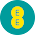Sycnhronizing Current

After proper synchronization of the alternators, they will run in synchronism. A synchronizing torque will be developed if any of the alternator drops out of synchronism and will bring it back to the synchronism.
Consider the two alternators shown in the Fig. 1 which are in exact synchronism. Due to this they are having same terminal p.d. and with reference to their local circuit they are in exact phase opposition. So there will not be any circulating current in the local circuit. The e.m.f. Eof alternator 1 is in exact phase opposition to that of alternator E2 .Fig. 1
With respect to external load, the e.m.f.s of the two alternators are in the same direction although they are in phase opposition with reference to local circuit. There will be no resultant voltage in the local circuit.
Now assume that speed of alternator 2 is changed such that its e.m.f. Efalls by an angle α. But Eand E2 are equal in magnitude. The resultant voltage in this case will cause a current in the local circuit which is called synchronizing current. This circulating current is given by,
ISY  = E/Z
where             Zs = Synchronous impedance of winding of alternator
The phase angle of ISY  is given by an angle θ which can be computed as tanθ = Xs/Ra where Xs is synchronous reactance and Ra is armature resistance. This angle is almost 90o.Fig. 2

Thus ISY  lags Er by almost 90o and approximately in phase with E1. This current is generating current with respect to alternator 1 since it is in the same direction as that of e.m.f. of alternator 1 while it will be motoring current for alternator 2 as it is in the opposite direction as that of e.m.f. of alternator 2. This current ISY will produce a synchronizing torque which will try to retard alternator 1 whereas accelerate the alternator 2.
The power output of alternator 1 supplies power input to alternator 2 and copper losses in the local path formed by armatures of two alternators.
Power output of alternator 1 = E1 ISY  cosΦ1
This power is approximately equal to E1 ISY  as Φ1 is small and is almost in phase with E1. This power is called synchronizing power. Similarly power input to alternator 2 is E2 ISY  cosΦ2 which is equal to E2 ISY  as Φ2 is also small.
E1 ISY  = E2 ISY  + Cu losses in the local circuit
Let               E1 = E2 =  E
Let the magnitude of resultant e.m.f. be Er which is given by,

But α is small. ...  sin α/2  = α/2
E = 2 E (α/2) = αE                                                   ............ ( θ = 90o , sin θ = 1)
The electrical angle α is expressed in radians.

Xs is synchronous reactance of each machine
Now, synchronizing power per phase,  PSY  =   E1 . ISY  =   E  . ISY

If Ra is not assumed as negligible then will not be so the above expression can be written in exact form as,

For 3 phases, total synchronizing power is given by,

The above expression is valid for two alternators connected in parallel and operating at no load.
Now let us consider the case of alternator connected to infinite bus bar (the concept of infinite bus bar is explained later) then the above expression for synchronizing power is still valid with the changes of reactance of only one alternators.
...           E = α E

If Ra is neglected,

The exact expression is,

For 3 phases, total synchronizing power

Now assuming that E2  has advanced in phase shown in the Fig. 2(b). The synchronizing current  ISY  in this case will be generating current for machine 2 and motoring current for machine 1. This will again produce a torque which will try to accelerate alternator 1 and try to retard alternator 2.
Note : Hence if synchronism between the two machines is lost then synchronizing current will flow in the local circuit which will produce a synchronizing torque.
This torque will tend to accelerate the lagging machine while will try to retard the leading machine. In case of machines which are loaded this current is superimposed on the load current.

1.2.